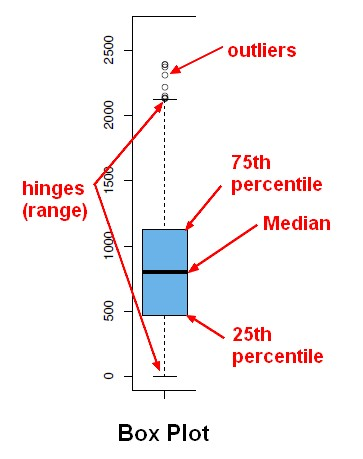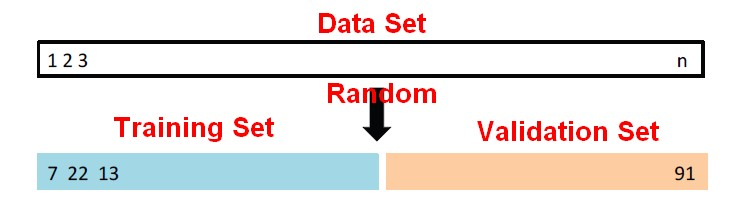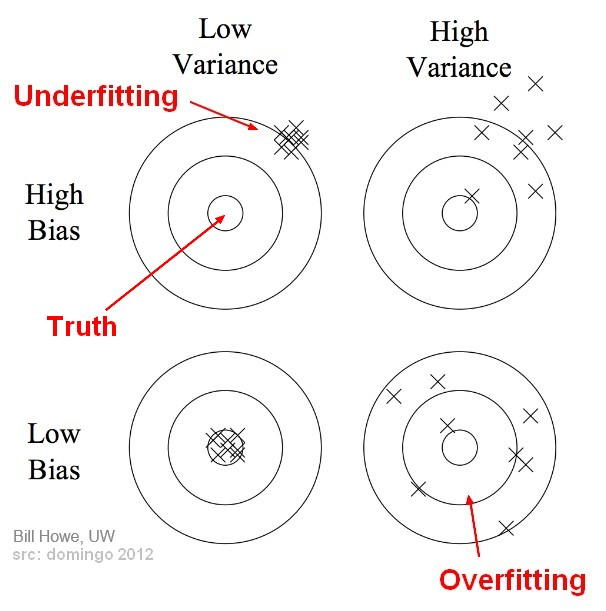# Statistics - ( Spread | Variability ) of a sample

An important element of a data set is how it is spread.

Variability is measure that describes the range and diversity of scores in a distribution

## Prediction

Recommended PagesData Visualization - Box Plot

A box plot is a good summary of a distribution and was invented by John Tukey. See Five-number summary The boxplot is a special case of the quantile function in that it only returns the 1st, 2nd and...Model Building - ReSampling Validation

Resampling method are a class of methods that estimate the test error by holding out a subset of the training set from the fitting process, and then applying the statistical learning method to those held...Process - Poisson Process

The Poisson process is a stochastic process in which events occur: continuously independently (of the time since the last event) - (ie random) at a constant / known average rate in a fixed interval...Statistical Learning - Two-fold validation

Two-fold validation is a resampling method. It randomly divides the available set of samples into two parts: a training set and a validation or hold-out set. The model is fit on the training set,...Statistics - (Data|Data Set) (Summary|Description) - Descriptive Statistics

Summary are a single value summarizing a array of data. They are: selected or calculated through reduction operations. They are an important element of descriptive analysis One of the most important...Statistics - (Variance|Dispersion|Mean Square) (MS)

The variance shows how widespread the individuals are from the average. The variance is how much that the estimate varies around its average. It's a measure of consistency. A very large variance means...Statistics - Deviation Score (for one observation)

The deviation score is the difference between two scores as : a value and an other value (usually the predicted score: mean, ...) See also: for a sample, for a measurement, (??) ...Statistics - Poisson Distribution

The Poisson distribution is a discrete probability distribution (of count) that expresses the probability of a given number of events (count) that will occur in an interval on a Poisson process Like the...Statistics - Range

The range of a data set is the difference between: the minimum (smallest) and the maximum (largest) value of this data set. It's the smallest interval which contains all the data. It's a measure...Statistics / Probability - Gaussian function ( )

In statistics and probability theory, Gaussian functions appear as the density function of the normal distribution, which is a limiting probability distribution of complicated sums, according to the central...